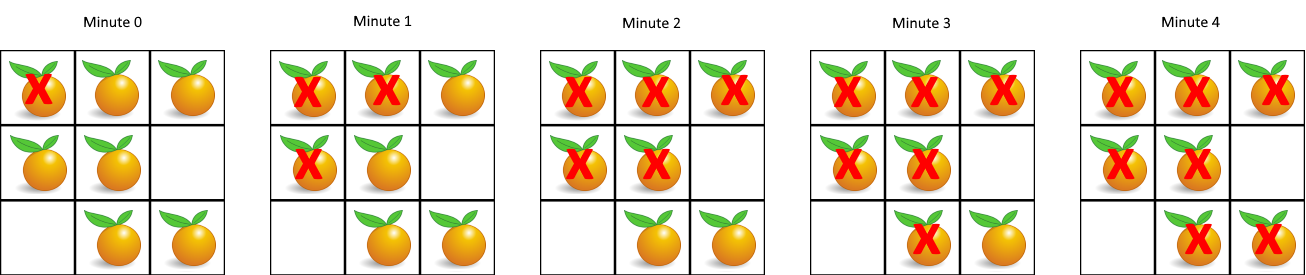# [Leetcode]994. Rotting Oranges

limelimejiwon·2022년 3월 21일
0

## Python으로 공부하는 코딩테스트

목록 보기
18/67## 📄 Description

You are given an m x n grid where each cell can have one of three values:

• 0 representing an empty cell,
• 1 representing a fresh orange, or
• 2 representing a rotten orange.
Every minute, any fresh orange that is 4-directionally adjacent to a rotten orange becomes rotten.

Return the minimum number of minutes that must elapse until no cell has a fresh orange. If this is impossible, return -1.

### Example 1:Input: grid = [[2,1,1],[1,1,0],[0,1,1]] Output: 4

### Example 2:

Input: grid = [[2,1,1],[0,1,1],[1,0,1]] Output: -1 Explanation: The orange in the bottom left corner (row 2, column 0) is never rotten, because rotting only happens 4-directionally.

### Example 3:

Input: grid = [[0,2]] Output: 0 Explanation: Since there are already no fresh oranges at minute 0, the answer is just 0.

## 💻 My Submission

class Solution:
def orangesRotting(self, grid: List[List[int]]) -> int:
R,C =len(grid), len(grid)
rotten=[]
# find rotten oranges
for i in range(R):
for j in range(C):
if grid[i][j]==2:
rotten.append((i,j))

minute=0
while rotten:
while rotten:
position=rotten.pop(0)
r,c=position,position
# check if its neighbor are fresh
if r+1<R and grid[r+1][c]==1:
grid[r+1][c]=2
if r-1>=0 and grid[r-1][c]==1:
grid[r-1][c]=2
if c+1<C and grid[r][c+1]==1:
grid[r][c+1]=2
if c-1>=0 and grid[r][c-1]==1:
grid[r][c-1]=2
# if all the adjacent cell got rotten, 1 minute pass
minute+=1

# If there is fresh orange left then return -1
for i in range(R):
for j in range(C):
if grid[i][j]==1:
return -1

return minute

## 💊 Better Solution

	def orangesRotting(self, grid):
''' Fresh and Rotten Hash Set to track fresh and rotten oranges'''
fresh = set()
rotten = set()

for i in range(len(grid)):
for j in range(len(grid)):
if grid[i][j] == 1:
elif grid[i][j] == 2:

minutes = 0
directions = [[0,1], [1,0], [-1,0], [0,-1]]

"""while fresh oranges exist"""
while fresh:
infected = set()
'''for every rotten orange, check if its neighbors are fresh'''
for orange in rotten:
i = int(orange)
j = int(orange)

for direction in directions:
nextI = i + direction
nextJ = j + direction

'''if fresh, remove from fresh and add it to infected'''
if (str(nextI) + str(nextJ)) in fresh:
fresh.remove(str(nextI)+str(nextJ))
return minutes